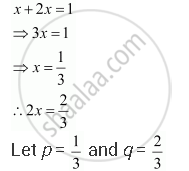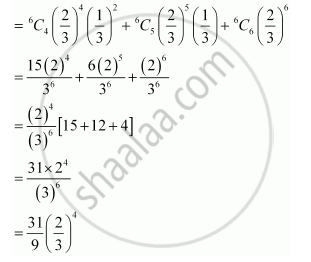Share

# An Experiment Succeeds Twice as Often as It Fails. Find the Probability that in the Next Six Trials, There Will Be at Least 4 Successes. - CBSE (Commerce) Class 12 - Mathematics

ConceptBernoulli Trials and Binomial Distribution

#### Question

An experiment succeeds twice as often as it fails. Find the probability that in the next six trials, there will be at least 4 successes.

#### Solution

The probability of success is twice the probability of failure.

Let the probability of failure be x.

∴ Probability of success = 2xLet X be the random variable that represents the number of successes in six trials.

By binomial distribution, we obtain

P (X = x) = ""^nC_x P^(n-x) q^x

Probability of at least 4 successes = P (X ≥ 4)

= P (X = 4) + P (X = 5) + P (X = 6)Is there an error in this question or solution?

#### Video TutorialsVIEW ALL 

Solution An Experiment Succeeds Twice as Often as It Fails. Find the Probability that in the Next Six Trials, There Will Be at Least 4 Successes. Concept: Bernoulli Trials and Binomial Distribution.
S